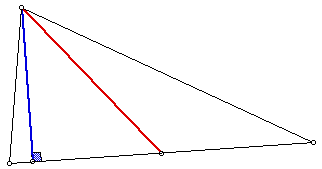# SAM

How many altitudes and medians are there in a triangle?In what type(s) of triangles is one altitude the same segment as a median? How many symmetry (folding or reflecting) lines can you draw in this type of triangle?

In what type(s) of triangles are all of the corresponding altitudes and mediansthe same? How many symmetry lines can you draw in this type of triangle?

Can exactly two of the altitudes of a triangle be the same segment as two medians? Why or why not?

Discuss the relationship between the number of symmetry lines in a triangle using altitudes and medians in your description.